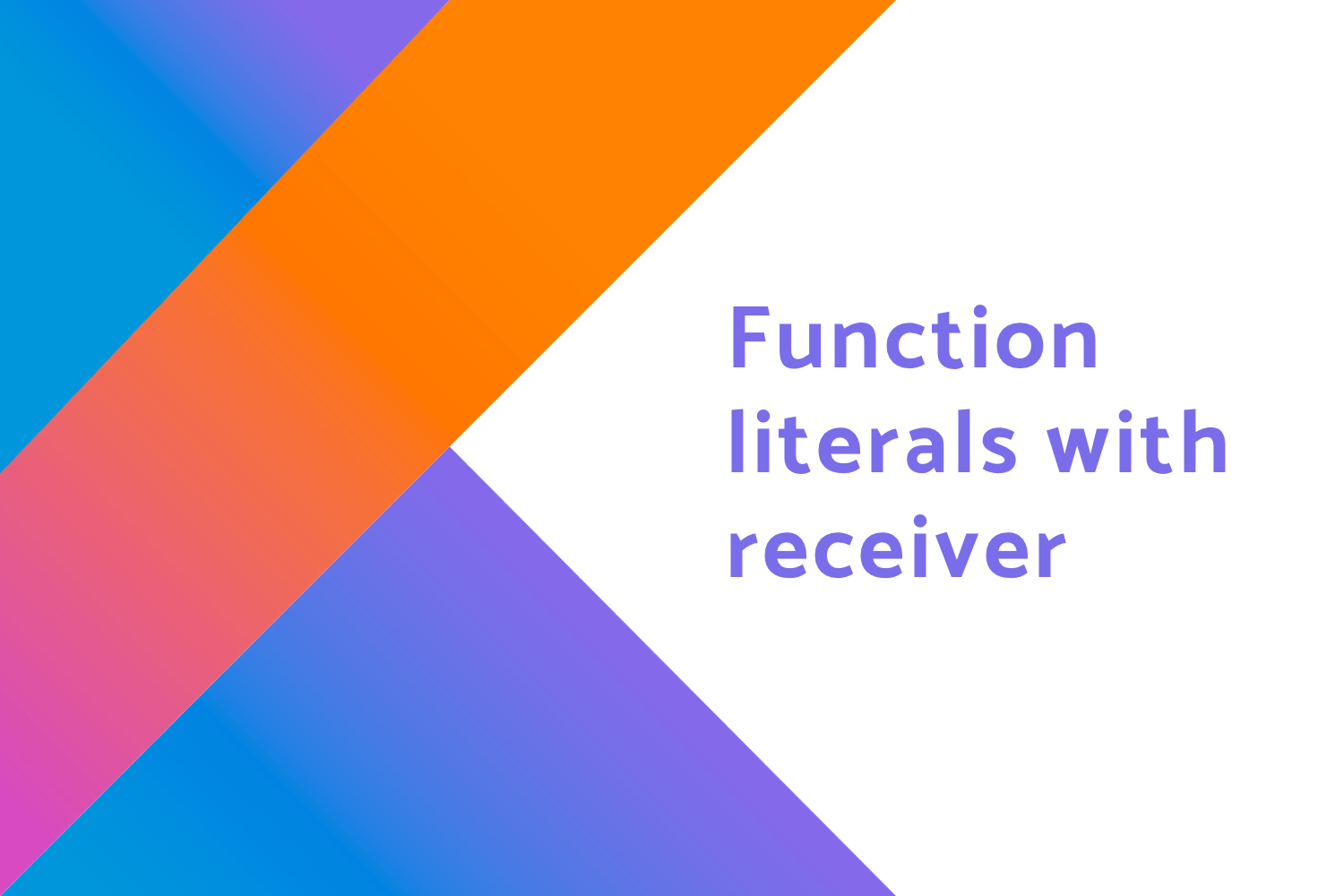# Function literals with receiver in KotlinIn this blog, we will learn about the function literals with receiver in kotlin. So before starting let's go through some basics.

First, let's check how to write a function. We will see how we can use a function and StringBuilder API for the task of creating a tag from given strings. The function looks like,

``````fun appendTag(string: String): String {
return StringBuilder().append("<")
.append(string)
.append(">")
.toString()
}``````

Now, let's say we have two strings MindOrks and AfterAcademy and want a to convert the strings to a tag then we call,

``````// Variables
val A = "MindOrks"

// Using the function
appendTag(A)
appendTag(B)``````

Here, we have to call the append function to print the output.

Now, in Kotlin to shorten this we have something called Extension Function. Let's create an extension function for creating the tag,

``````fun String.appendTag() {
StringBuilder().append("<")
.append(this)
.append(">")
.toString()
}``````

And, we use the extension functions in Kotlin like,

``````// Variables
val A = "MindOrks"

// Using the extension function
A.appendTag()
B.appendTag()``````

Here, you can see we are not calling the appendTag function again and again. Rather, we just call appendTag as an extension with the dot operator.

Both by calling the function and extension function we get the following output,

``````<MindOrks>

Now, we have successfully converted the strings to a tag using Extension function.

Now, we have to learn about Lambda and Higher Order function. For that, I am not going to explain here. You can learn all about lambdas and extension here.

Now, let's create a higher-order function and pass a lambda as a parameter.

We will create a function called buildString and pass StringBuilder as a parameter. It will be,

``````fun buildString(action: (StringBuilder) -> Unit): String {
val builder = StringBuilder()
action(builder)
return builder.toString()
}``````

Here,

• buildString takes a function action as a parameter
• action function takes StringBuilder (builder) as a parameter which returns Unit (void in Java)
• and the overall function buildString returns string as output.

Now, to use the function buildString, we call it using

``````buildString { builder ->
builder.append("<")
builder.append("MindOrks")
builder.append(">")
}``````

We are getting builder as a parameter because we are passing in action(builder)

Here, we are calling the buildString function as passing the builder as a parameter for the function.

So, to explain it better in simple terms let me expand the function.

``````buildString (fun someFunctionName(builder:StringBuilder) {
builder.append("<")
builder.append("MindOrks")
builder.append(">")
})``````

Here, we have passed a function to the buildString function having a parameter of type StringBuilder . Then we are calling the parameter builder to do the append operation for us as expected.

Note: This is just for explanatory purpose.

Now, when we remove the function and want to use lambda then we write the following,

``````buildString { builder ->
builder.append("<")
builder.append("MindOrks")
builder.append(">")
}``````

Where we just write the part which we wrote under the someFunctionName function. Here, the builder is a receiver

The receiver is used in kotlin to access the property of the receiver type without any additional line of code or qualifier

Now, to make life simpler we will use Idiomatic Kotlin. In that, we will remove the explicit dependency of the builder in lambda.

Now, let's take the buildString lambda,

``````buildString { builder ->
builder.append("<")
builder.append("MindOrks")
builder.append(">")
}``````

In this, we want to achieve is,

``````buildString {
append("<")
append("MindOrks")
append(">")
}``````

Here, we can access the builder using this keyword and while writing we can ignore this .

To explain how we can do it, let me expand the buildString lambda again,

``````buildString (fun someFunctionName(builder:StringBuilder) {
builder.append("<")
builder.append("MindOrks")
builder.append(">")
})``````
Note: This is just for explanatory purpose.

Now, to access the property of StringBuilder , we will create an extension function of it. Check above code samples to see how we create extension functions. To do it we will add,

``````buildString (fun someFunctionName(builder:StringBuilder).extFn() {
builder.append("<")
builder.append("MindOrks")
builder.append(">")
})``````
Note: This is just for explanatory purpose.

And since now we have the reference of StringBuilder in the function as we know it from above Extension function example. we can write the buildString as,

``````buildString (fun someFunctionName(builder:StringBuilder).extFn() {
append("<")
append("MindOrks")
append(">")
})``````

Now, we can also remove extFn() as,

``````buildString (fun someFunctionName(builder:StringBuilder).() {
append("<")
append("MindOrks")
append(">")
})``````

Here, you can see we removed extFn ().

and finally, we can remove the dummy explanatory function someFucntionName and get the desired output,

``````buildString {
append("<")
append("MindOrks")
append(">")
}``````

Here, you can see that we are not using the builder explicitly. Here it gives the reference of StringBuilder in the lambda. We can also call the properties of StringBuilder by using this as well.

Now, since we are getting an extension function we need to replace the buildString function with the following,

``````fun buildString(action: (StringBuilder).() -> Unit): String {
val stringBuilder = StringBuilder()
action(stringBuilder)
return stringBuilder.toString()
}``````

Here, you can see only one difference, we changed the code from (StringBuilder) -> Unit to (StringBuilder) .() -> Unit .

If you remember from the above code of creating an extension function, you would notice we are just creating basically a function of type ``` () -> Unit ``` with ``` StringBuilder ``` as the receiver without explicitly specifying a name.

So this is how you can create a lambda with a receiver. These are useful in creating DSLs in Kotlin.

#### Misc

In your Android project, you might be using lambda with the receiver without knowing it. If you have used apply , you would be already using it. The code for apply looks like,

``````public inline fun <T> T.apply(block: T.() -> Unit): T {

block()
return this
}``````

Here, you can see it takes a function block with a receiver type of Generic. and when we use it we write,

``````textView.apply {
text = "MindOrks"
setOnClickListener {

}
}``````

Here as well we use the property of TextView in the lambda. This is possible because of the receiver in the lambda.

Happy learning

Team MindOrks :)